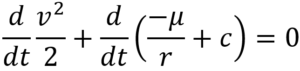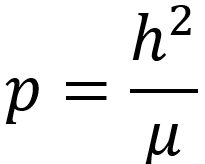# Specific Energy for a Two-Body Orbit

Specific energy for a two-body orbit will be derived below. Specific energy of an orbit is constant in the two-body system. To further refine our definition, we are concerned with conservative forces only. This way specific mechanical energy is an exchange between potential and kinetic energy without drag or other perturbation losses that are non-conservative.

Specific energy was provided without proof in Eq. (1), from the previous article, "Orbital Speed for All Conic Sections," reproduced below.(1)

Specific energy is further reduced for all conic sections in Eq. (2) as a function of the gravitational parameter for the central body and the semimajor axis of the orbit.(2)

## Specific Energy Derivation

Given the two-body equation of motion, Eq. (3) we derive the specific energy for all conic orbits.(3)

### Step 1: Multiply by r-dot

Rearranging and multiplying by the derivative of position with respect to time,### Step 2: Replace with derivatives of KE and PE w/r to time

As the scalar velocity multiplied by the scalar derivative of velocity term is equivalent to the derivative of kinetic energy with respect to time, we replace it as shown below on the left-hand side. The derivative of potential energy or the gravitational parameter over the radial distance is equivalent to the gravitational parameter over the radial distance squared multiplied by the scalar derivative of the position. To make the term general, we include the constant, c, which physically represents where we draw the datum for potential energy.### Step 3: Set the reference for PE to 0 (reference level at infinity)

Setting the constant, c, to zero is equivalent to setting the datum for potential energy at infinity.(1)

## Function of semimajor axis and gravitational parameter

Using the periapsis and semilatus rectum's relationship to the specific angular momentum and gravitational parameter, the specific energy can be reduced to Eq. (2).(2)

The semimajor axis is positive for circular and elliptic orbits, infinite for a parabolic orbit, and negative for a hyperbolic orbit. Thus, the energy of each is negative, zero, and positive, respectively.